# Learning Electronics

Learn to build electronic circuits

# Voltmeter design

### Question 1:

Suppose I were about to measure an unknown voltage with a manual-range voltmeter. This particular voltmeter has several different voltage measurement ranges to choose from:

500 volts
250 volts
100 volts
50 volts
25 volts
10 volts
5 volts

What range would be best to begin with, when first measuring this unknown voltage with the meter? Explain your answer.
Begin by setting the voltmeter to its highest range: 500 volts. Then, see if the movement needle registers anything with the meter leads connected to the circuit. Decide to change the meter's range based on this first indication.

Notes:
I always like to have my students begin their test equipment familiarity by using old-fashioned analog multimeters. Only after they have learned to be proficient with an inexpensive meter do I allow them to use anything better (digital, auto-ranging) in their work. This forces students to appreciate what a "fancy" meter does for them, as well as teach them basic principles of instrument ranging and measurement precision.

### Question 2:

What would happen to this meter movement, if connected directly to a 6-volt battery?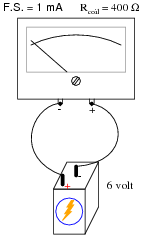Two things would happen: first, the movement would most likely be damaged from excessive current. Secondly, the needle would move to the left instead of the right (as it normally should), because the polarity is backward.

Notes:
When an electromechanical meter movement is overpowered, causing the needle to ßlam" all the way to one extreme end of motion, it is commonly referred to as "pegging" the meter. I've seen meter movements that have been "pegged" so badly that the needles are bent from hitting the stop!
Based on your students knowledge of meter movement design, ask them to tell you what they think might become damaged in a severe over-power incident such as this. Tell them to be specific in their answers.

### Question 3:

An important step in building any analog voltmeter or ammeter is to accurately determine the coil resistance of the meter movement. In electrical metrology, it is often easier to obtain extremely precise (ßtandard") resistance values than it is to obtain equally precise voltage or current measurements. One technique that may be used to determine the coil resistance of a meter movement without need to accurately measure voltage or current is as follows.
First, connect a decade box type of variable resistance in series with a regulated DC power supply, then to the meter movement to be tested. Adjust the decade box's resistance so that the meter movement moves to some precise point on its scale, preferably the full-scale (100%) mark. Record the decade box's resistance setting as R1: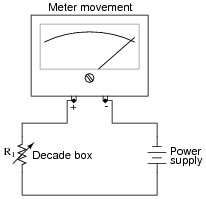Then, connect a known resistance in parallel with the meter movement's terminals. This resistance will be known as Rs, the shunt resistance. The meter movement deflection will decrease when you do this. Re-adjust the decade box's resistance until the meter movement deflection returns to its former place. Record the decade box's resistance setting as R2: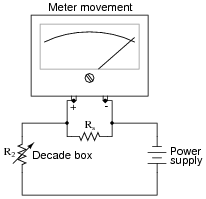The meter movement's coil resistance (Rcoil) may be calculated following this formula:

 Rcoil = Rs R2 (R1 - R2)

Your task is to show where this formula comes from, deriving it from Ohm's Law and whatever other equations you may be familiar with for circuit analysis.
Hint: in both cases (decade box set to R1 and set to R2), the voltage across the meter movement's coil resistance is the same, the current through the meter movement is the same, and the power supply voltage is the same.
One place to start from is the voltage divider equation, VR = VT ([R/(RT)] ) applied to each circuit scenario:

 Vmeter = Rcoil R1 + Rcoil

 Vmeter = Rcoil || Rs R2 + (Rcoil || Rs)

Since we know that the meter's voltage is the same in the two scenarios, we may set these equations equal to each other:

 Rcoil R1 + Rcoil = Rcoil || Rs R2 + (Rcoil || Rs)

Note: the double-bars in the above equation represent the parallel equivalent of Rcoil and Rs, for which you will have the substitute the appropriate mathematical expression.

Notes:
This problem is really nothing more than an exercise in algebra, although it also serves to show how precision electrical measurements may be obtained by using standard resistors rather than precise voltmeters or ammeters.

### Question 4:

 Don't just sit there! Build something!!

Learning to mathematically analyze circuits requires much study and practice. Typically, students practice by working through lots of sample problems and checking their answers against those provided by the textbook or the instructor. While this is good, there is a much better way.
You will learn much more by actually building and analyzing real circuits, letting your test equipment provide the änswers" instead of a book or another person. For successful circuit-building exercises, follow these steps:

1.
Carefully measure and record all component values prior to circuit construction.
2.
Draw the schematic diagram for the circuit to be analyzed.
3.
Carefully build this circuit on a breadboard or other convenient medium.
4.
Check the accuracy of the circuit's construction, following each wire to each connection point, and verifying these elements one-by-one on the diagram.
5.
Mathematically analyze the circuit, solving for all values of voltage, current, etc.
6.
Carefully measure those quantities, to verify the accuracy of your analysis.
7.
If there are any substantial errors (greater than a few percent), carefully check your circuit's construction against the diagram, then carefully re-calculate the values and re-measure.

Avoid very high and very low resistor values, to avoid measurement errors caused by meter "loading". I recommend resistors between 1 kW and 100 kW, unless, of course, the purpose of the circuit is to illustrate the effects of meter loading!
One way you can save time and reduce the possibility of error is to begin with a very simple circuit and incrementally add components to increase its complexity after each analysis, rather than building a whole new circuit for each practice problem. Another time-saving technique is to re-use the same components in a variety of different circuit configurations. This way, you won't have to measure any component's value more than once.
Let the electrons themselves give you the answers to your own "practice problems"!

Notes:
It has been my experience that students require much practice with circuit analysis to become proficient. To this end, instructors usually provide their students with lots of practice problems to work through, and provide answers for students to check their work against. While this approach makes students proficient in circuit theory, it fails to fully educate them.
Students don't just need mathematical practice. They also need real, hands-on practice building circuits and using test equipment. So, I suggest the following alternative approach: students should build their own "practice problems" with real components, and try to mathematically predict the various voltage and current values. This way, the mathematical theory "comes alive," and students gain practical proficiency they wouldn't gain merely by solving equations.
Another reason for following this method of practice is to teach students scientific method: the process of testing a hypothesis (in this case, mathematical predictions) by performing a real experiment. Students will also develop real troubleshooting skills as they occasionally make circuit construction errors.
Spend a few moments of time with your class to review some of the "rules" for building circuits before they begin. Discuss these issues with your students in the same Socratic manner you would normally discuss the worksheet questions, rather than simply telling them what they should and should not do. I never cease to be amazed at how poorly students grasp instructions when presented in a typical lecture (instructor monologue) format!
A note to those instructors who may complain about the "wasted" time required to have students build real circuits instead of just mathematically analyzing theoretical circuits:
What is the purpose of students taking your course?
If your students will be working with real circuits, then they should learn on real circuits whenever possible. If your goal is to educate theoretical physicists, then stick with abstract analysis, by all means! But most of us plan for our students to do something in the real world with the education we give them. The "wasted" time spent building real circuits will pay huge dividends when it comes time for them to apply their knowledge to practical problems.
Furthermore, having students build their own practice problems teaches them how to perform primary research, thus empowering them to continue their electrical/electronics education autonomously.
In most sciences, realistic experiments are much more difficult and expensive to set up than electrical circuits. Nuclear physics, biology, geology, and chemistry professors would just love to be able to have their students apply advanced mathematics to real experiments posing no safety hazard and costing less than a textbook. They can't, but you can. Exploit the convenience inherent to your science, and get those students of yours practicing their math on lots of real circuits!

### Question 5:

What is a galvanometer? How might you build your own galvanometer from commonly available components?
There are several sources of information on galvanometers, both historical and modern. I leave it to you to do the research and present your findings.

Notes:
It is possible to make a crude galvanometer from a large audio speaker, using the voice coil/cone assembly as the moving element. Using a small laser and a mirror, it should be easy to construct a light-beam galvanometer, for greater sensitivity. This could be a fun and educational classroom experiment!

### Question 6:

Describe the design and function of a PMMC style meter movement.
"PMMC" is an acronym standing for "Permanent Magnet, Moving Coil". In essence, a PMMC meter movement is built like a small DC electric motor, with limited range of motion.

Notes:
Many textbooks provide good illustrations of PMMC meter movements. Your students may find some electronic images of PMMC meter movements on the internet. If possible, have a video projector in the classroom for projecting images like this that your students download.

### Question 7:

We know that connecting a sensitive meter movement directly across the terminals of a substantial voltage source (such as a battery) is a Bad Thing. So, I want you to determine what other component(s) must be connected to the meter movement to limit the current through its coil, so that connecting the circuit to a 6-volt battery results in the meter's needle moving exactly to the full-scale position: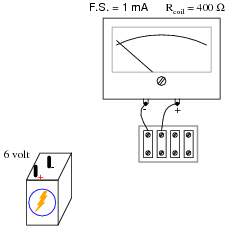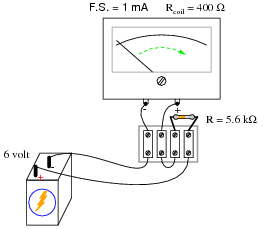Notes:
Beginning students sometimes feel "lost" when trying to answer a question like this. They may know how to apply Ohm's Law to a circuit, but they do not know how to design a circuit that makes use of Ohm's Law for a specific purpose. If this is the case, you may direct their understanding through a series of questions such as this:

Why does the meter movement "peg" if directly connected to the battery?
What type of electrical component is good at limiting current?
How might we connect this component to the meter (series or parallel)? (Draw both configurations and let the student determine for themselves which connection pattern fulfills the goal of limiting current to the meter.)

The math is simple enough in this question to allow solution without the use of a calculator. Whenever possible, I challenge students during discussion time to perform any necessary arithmetic "mentally" (i.e. without using a calculator), even if only to estimate the answer. I find many American high school graduates unable to do even very simple arithmetic without a calculator, and this lack of skill causes them no small amount of trouble. Not only are these students helpless without a calculator, but they lack the ability to mentally check their calculator-derived answers, so when they do use a calculator they have no idea whether their answer is even close to being correct.

### Question 8:

Calculate the necessary resistance value and power rating for Rrange in order to make the meter movement respond as a voltmeter with a range of 0 to 100 volts: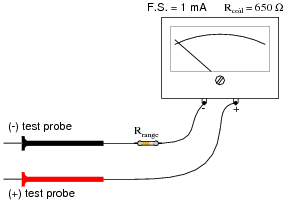Rrange = 99.35 k W, [1/8] watt will be sufficient.

Notes:
This is really nothing more than a simple series circuit problem, although the context of it being a voltmeter seems to confuse some students. If you find a large percentage of your class not understanding where to begin in a problem such as this, it means they really don't understand series circuits - all they learned to do when studying series resistor circuits before is to follow an easy sequence of steps to find voltages and currents in series resistor circuits. They did not learn the concepts well enough to abstract to something that looks just a little bit different.

### Question 9:

Calculate the necessary resistance value and power rating for Rrange in order to make the meter movement respond as a voltmeter with a range of 0 to 50 volts: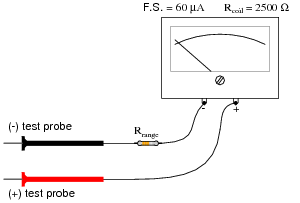Rrange = 830.83 k W, [1/8] watt will be sufficient.

Notes:
This is really nothing more than a simple series circuit problem, although the context of it being a voltmeter seems to confuse some students. If you find a large percentage of your class not understanding where to begin in a problem such as this, it means they really don't understand series circuits - all they learned to do when studying series resistor circuits before is to follow an easy sequence of steps to find voltages and currents in series resistor circuits. They did not learn the concepts well enough to abstract to something that looks just a little bit different.

### Question 10:

Calculate the necessary resistance values to give this multi-range voltmeter the ranges indicated by the selector switch positions: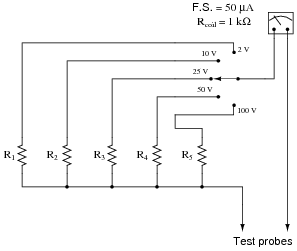R1 = 39 k W
R2 = 199 k W
R3 = 499 k W
R4 = 999 k W
R5 = 1.999 M W

Notes:
This is really nothing more than a set of simple series circuit problems, although the context of it being a voltmeter seems to confuse some students. If you find a large percentage of your class not understanding where to begin in a problem such as this, it means they really don't understand series circuits - all they learned to do when studying series resistor circuits before is to follow an easy sequence of steps to find voltages and currents in series resistor circuits. They did not learn the concepts well enough to abstract to something that looks just a little bit different.

### Question 11:

Calculate the necessary resistance values to give this multi-range voltmeter the ranges indicated by the selector switch positions: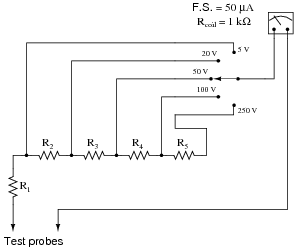R1 = 99 k W
R2 = 300 k W
R3 = 600 k W
R4 = 1 M W
R5 = 3 M W
Hint: if you need help getting started in this problem, begin with calculating the value of R1.

Notes:
This is really nothing more than a set of simple series circuit problems, although the context of it being a voltmeter seems to confuse some students. If you find a large percentage of your class not understanding where to begin in a problem such as this, it means they really don't understand series circuits - all they learned to do when studying series resistor circuits before is to follow an easy sequence of steps to find voltages and currents in series resistor circuits. They did not learn the concepts well enough to abstract to something that looks just a little bit different.
You should point out to your students how the series arrangement of the range resistors lends itself to more common resistance values, as opposed to having a separate range resistor for each range. There is a downside to this design, however: reliability. Discuss with your students the consequences of öpen" resistor faults in both types of voltmeter designs.

### Question 12:

Ideally, should a voltmeter have a very low input resistance, or a very high input resistance? (Input resistance being the amount of electrical resistance intrinsic to the meter, as measured between its test leads.) Explain your answer.
Ideally, a voltmeter should have the greatest amount of input resistance possible. This is important when using it to measure voltage sources and voltage drops in circuits containing large amounts of resistance.

Notes:
The answer to this question is related to the very important principle of meter loading. Technicians, especially, have to be very aware of meter loading, and how erroneous measurements may result from it. The answer is also related to how voltmeters are connected with the circuits under test: always in parallel!

### Question 13:

Explain what the ohms-per-volt sensitivity rating of an analog voltmeter means. Many analog voltmeters exhibit a sensitivity of 20 kW per volt. Is it better for a voltmeter to have a high ohms-per-volt rating, or a low ohms-per-volt rating? Why?
The öhms-per-volt" sensitivity rating of a voltmeter is an expression of how many ohms of input resistance the meter has, per range of volt measurement. The higher this figure is, the better the voltmeter.

Notes:
If students have analog voltmeters in their possession (which I greatly encourage them to have), the ohms-per-volt sensitivity rating is often found in a corner of the meter scale, in fine print. If not, the rating should be found in the user's guide that came with the meter.

### Question 14:

Fundamentally, what single factor in a voltmeter's design establishes its ohms-per-volt sensitivity rating?
If your answer is, "the value of the series resistor(s)," you are incorrect.

Notes:
Students' immediate impression is that the range resistor value must establish the sensitivity rating, because they see the resistor as having the most impact on input resistance. However, some quick calculations with different range resistor values prove otherwise! Meter sensitivity is independent of any series-connected range resistor values.
You might want to ask your students why meter movement coil resistance is not a factor in determining voltmeter sensitivity. Challenge your students with setting up sample circuit problems to prove the irrelevance of coil resistance on voltmeter sensitivity. Let them figure out how to set up the problems, rather than you setting up the problems for them!

### Question 15:

Determine the different range values of this multi-range voltmeter: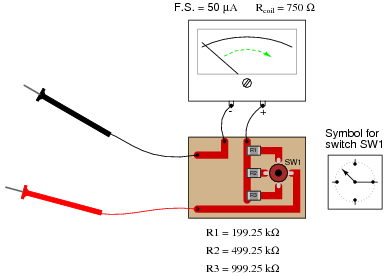All components on the printed circuit board are ßurface-mount," soldered onto the top surfaces of the copper traces. The switch (SW1) schematic diagram is shown to the immediate right of the circuit board, with resistor values shown below the circuit board.
Ranges = 10 V, 25 V, and 50 V.

Notes:
Determining the voltage ranges for this voltmeter is simply an exercise in Ohm's Law. The arithmetic is simple enough to permit solution without the use of calculators, so challenge your students during discussion time to work through the math "the old-fashioned way".

### Question 16:

What if this voltmeter suddenly stopped working when set in its middle range. The upper and lower ranges still function just fine, though. Identify the most likely source of the problem.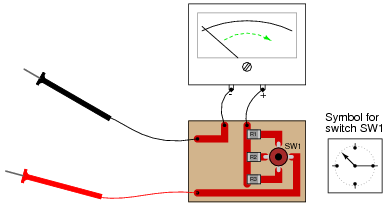The middle contact in switch SW2 is open. This, despite being the most likely failure, is not the only possible failure that could cause this problem (middle range not functioning)!
Challenge question: explain how you could verify the nature of the fault without using another meter.

Notes:
Brainstorm some other alternative possibilities for causing the problem, along with diagnostic procedures to verify each one of them (using another meter, if necessary). Then, discuss with your students the reason why a switch failure is more likely than any of the other faults.

### Question 17:

Suppose you tried to measure the voltage at test point 2 (TP2) with a digital voltmeter having an input resistance of 10 MW. How much voltage would it indicate? How much voltage should it ideally indicate?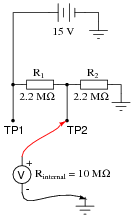Ideally, of course, this voltage divider circuit should exhibit 7.5 volts at test point 2. The voltmeter, however, will register only 6.76 volts.
Follow-up question: is the voltmeter registering inaccurately, or is its connection to the circuit actually changing VTP2? In other words, what is the actual voltage at TP2 with the voltmeter connected as shown?

Notes:
An analogy I often use to explain meter loading is the use of a pressure gauge to measure the air pressure in a pneumatic tire. In order to measure the pressure, some of the air must be let out of the tire, which of course changes the tire's air pressure.
And in case you are wondering: no, this is not an example of Heisenberg's Uncertainty Principle, popularly misunderstood as error introduced by measurement. The Uncertainty Principle is far more profound than this!

### Question 18:

Suppose you tried to measure the voltage at all three test points with an analog voltmeter having a sensitivity rating of 20 kW per volt, set on the 10 volt scale. How much voltage would it indicate at each test point? How much voltage should it ideally indicate at each test point?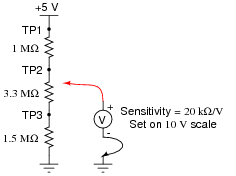Test point Ideal voltage Meter indication TP1 TP2 TP3

 Test point Ideal voltage Meter indication TP1 5 V 5 V TP2 4.138 V 0.805 V TP3 1.293 V 0.197 V

Notes:
An analogy I often use to explain meter loading is the use of a pressure gauge to measure the air pressure in a pneumatic tire. In order to measure the pressure, some of the air must be let out of the tire, which of course changes the tire's air pressure.
And in case you are wondering: no, this is not an example of Heisenberg's Uncertainty Principle, popularly misunderstood as error introduced by measurement. The Uncertainty Principle is far more profound than this!